Win up to 100% scholarship on Aakash BYJU'S JEE/NEET courses with ABNAT Win up to 100% scholarship on Aakash BYJU'S JEE/NEET courses with ABNAT

# JEE Main 2022 June 29 – Shift 1 Physics Question Paper with Solutions

The JEE Main 2022 June 29 – Shift 1 Physics Question Paper with Solutions is provided here for the benefit of students. The JEE 2022 question paper is prepared based on the physics curriculum for Classes XI and XII. Our subject experts have answered the questions precisely and accurately. Students will be better able to understand the topics covered in the question paper, and will be able to grasp the concepts quickly. It will also help them gain a deeper understanding of the Physics exam’s structure, question types, and other important aspects of the question paper.

## JEE Main 2022 June 29th Physics Shift 1 Question Paper and Solutions

#### SECTION – A

Multiple Choice Questions: This section contains 20 multiple choice questions. Each question has 4 choices (1), (2), (3) and (4), out of which ONLY ONE is correct.

1. Two balls A and B are placed at the top of 180 m tall tower. Ball A is released from the top at t = 0 s. Ball B is thrown vertically down with an initial velocity u at t = 2 s. After a certain time, both balls meet 100 m above the ground. Find the value of u in ms–1 [use g = 10 ms–2]

(A) 10

(B) 15

(C) 20

(D) 30

Sol. Let us assume that they meet at t = t0

$$\begin{array}{l}A:80=\frac{1}{2}gt_0^2….(i)\end{array}$$
$$\begin{array}{l}B: 80=u\left (t_0-2 \right )+\frac{1}{2}g\left ( t_0-2 \right )^2….(ii)\end{array}$$

From (i), t0 = 4

⇒ 80 = 2u + 5(2)2

u= 30 m/s

2. A body of mass M at rest explodes into three pieces, in the ratio of masses 1 : 1 : 2. Two smaller pieces fly off perpendicular to each other with velocities of 30 ms–1 and 40 ms–1 respectively. The velocity of the third piece will be

(A) 15 ms–1

(B) 25 ms–1

(C) 35 ms–1

(D) 50 ms–1

Sol. Conserving momentum:

$$\begin{array}{l}m\left ( 30\hat{i} \right )+m\left ( 40\hat{j}\right )+2m\left ( \vec{v} \right)=\vec{0}\end{array}$$
$$\begin{array}{l}\Rightarrow \vec{v}=-15\hat{i}-20\hat{j}\end{array}$$
$$\begin{array}{l}\Rightarrow \left|\vec{v} \right| =25~\text{m/s}\end{array}$$

3. The activity of a radioactive material is 2.56 × 10–3 Ci. If the half life of the material is 5 days, after how many days the activity will become 2 × 10–5 Ci?

(A) 30 days

(B) 35 days

(C) 40 days

(D) 25 days

Sol.

$$\begin{array}{l}A=A_0e^{-\lambda t}\end{array}$$

2 × 10–5 = 2.56 × 10–3e–λt

$$\begin{array}{l}\Rightarrow e^{-\lambda t}=\frac{1}{128}=\left [ \frac{1}{2} \right ]^7\end{array}$$
$$\begin{array}{l}\Rightarrow t=7t_\frac{1}{2}=35~~\textup{days}\end{array}$$

4. A spherical shell of 1 kg mass and radius R is rolling with angular speed ω on horizontal plane (as shown in figure). The magnitude of angular momentum of the shell about the origin O is

$$\begin{array}{l}\frac{a}{3}R^2\omega\ \text{. The value of a will be}\end{array}$$(A) 2

(B) 3

(C) 5

(D) 4

Sol.

$$\begin{array}{l}\vec{L_0}=\vec{L}_\textup{of cm}+\vec{L}_\textup{about cm}\end{array}$$
$$\begin{array}{l}\Rightarrow \frac{a}{3}R^2\omega=mvR+\frac{2}{3}mR^2\omega=\frac{5}{3}mR^2\omega \end{array}$$

a = 5

5. A cylinder of fixed capacity of 44.8 litres contains helium gas at standard temperature and pressure. The amount of heat needed to raise the temperature of gas in the cylinder by 20.0°C will be

(Given gas constant R = 8.3 JK–1-mol–1)

(A) 249 J

(B) 415 J

(C) 498 J

(D) 830 J

Sol. ΔQ = nCvΔT (Isochoric process)

$$\begin{array}{l}=2\times\frac{3R}{2}\times20 \end{array}$$

= 498 J

6. A wire of length L is hanging from a fixed support. The length changes to L1 and L2 when masses 1 kg and 2 kg are suspended, respectively, from its free end. Then the value of L is equal to

$$\begin{array}{l}(A)\ \sqrt{L_1L_2} \end{array}$$
$$\begin{array}{l}(B)\ \frac{L_1+L_2}{2}\end{array}$$

(C) 2L1L2

(D) 3L1 – 2L2

Sol.

$$\begin{array}{l}y=\frac{FL}{A\Delta L}\end{array}$$
$$\begin{array}{l}\Rightarrow \Delta L=\frac{FL}{Ay}\end{array}$$
$$\begin{array}{l}\Rightarrow L_1=L+\frac{\left ( 1g \right )L}{Ay}….(i) \end{array}$$

and

$$\begin{array}{l}L_2=L+\frac{\left ( 2g \right )L}{Ay} ….(ii) \end{array}$$

L = 2L1L2

7. Given below are two statements : one is labelled as AssertionA and the other is labelled as ReasonR.

Assertion A : The photoelectric effect does not takes place, if the energy of the incident radiation is less than the work function of a metal.

Reason R :Kinetic energy of the photoelectrons is zero, if the energy of the incident radiation is equal to the work function of a metal.

In the light of the above statements, choose the most appropriate answer from the options given below.

(A) Both A and R are correct and R is the correct explanation of A

(B) Both A and R are correct but R is not the correct explanation of A

(C) A is correct but R is not correct

(D) A is not correct but R is correct

Sol. When energy of incident radiation is equal to the work function of the metal, then the KE of photoelectrons would be zero. But this statement does not comment on the situation when energy is less than the work function.

8. A particle of mass 500 gm is moving in a straight line with velocity v = bx5/2. The work done by the net force during its displacement from x = 0 to x = 4 m is : (Take b = 0.25 m–3/2s–1).

(A) 2 J

(B) 4 J

(C) 8 J

(D) 16 J

Sol. Wtotal = ΔK

$$\begin{array}{l}=\frac{1}{2}\left ( \frac{1}{2} \right )\left [ \left\{b\left ( 4 \right )^{5/2} \right\}^2-0 \right ]\end{array}$$
$$\begin{array}{l}=\frac{b^2}{4}\times4^5 \end{array}$$

Wtotal = 16 J

9. A charge particle moves along circular path in a uniform magnetic field in a cyclotron. The kinetic energy of the charge particle increases to 4 times its initial value. What will be the ratio of new radius to the original radius of circular path of the charge particle

(A) 1 : 1

(B) 1 : 2

(C) 2 : 1

(D) 1 : 4

Sol.

$$\begin{array}{l}R=\frac{mv}{Bq}=\frac{\sqrt{2mk}}{Bq}\end{array}$$
$$\begin{array}{l}\Rightarrow R\propto\sqrt{K}\end{array}$$

⇒ ratio = 2 : 1

10. For a series LCR circuit, I vs ω curve is shown :

(a) To the left of ωr, the circuit is mainly capacitive.

(b) To the left of ωr, the circuit is mainly inductive.

(c) At ωr, impedance of the circuit is equal to the resistance of the circuit.

(d) At ωr, impedance of the circuit is 0.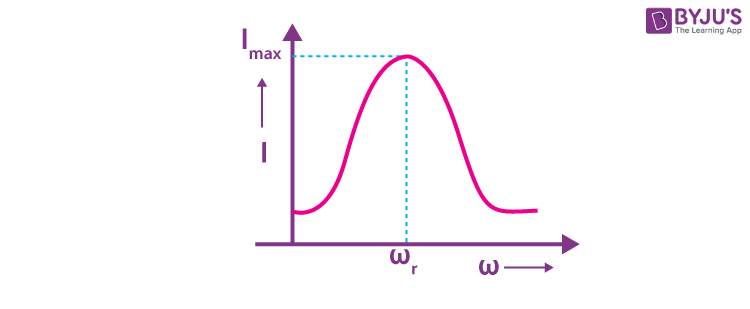Choose the most appropriate answer from the options given below.

(A) (a) and (d) only

(B) (b) and (d) only

(C) (a) and (c) only

(D) (b) and (c) only

Sol. We know that

$$\begin{array}{l}X_c=\frac{1}{\omega C} \end{array}$$
and XL = ωL

Also, at ω = ωr : XL = XC

⇒ For ω<ωr : capacitive

and At

$$\begin{array}{l}\omega = \omega_r:z=\sqrt{R^2+\left ( X_L-X_C \right )^2} =R\end{array}$$

11. A block of metal weighing 2 kg is resting on a frictionless plane (as shown in figure). It is struck by a jet releasing water at a rate of 1 kgs–1 and at a speed of 10 ms–1. Then, the initial acceleration of the block, in ms–2, will be: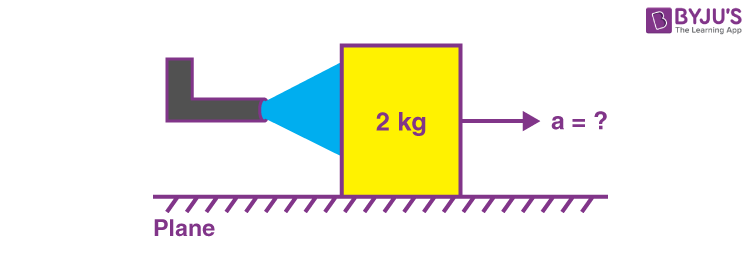(A) 3

(B) 6

(C) 5

(D) 4

Sol. F = ρv2a

⇒ 10 × 1 = 2 × acceleration

⇒ Acc. = 5 m/s2

12. In van der Wall equation

$$\begin{array}{l}\left [P+\frac{a}{V^2} \right ]\left [V-b \right ]=RT;\end{array}$$
P is pressure, V is volume, R is universal gas constant and T is temperature. The ratio of constants a/b is dimensionally equal to:

$$\begin{array}{l}(A)\ \frac{P}{V} \end{array}$$
$$\begin{array}{l}(B)\ \frac{V}{P}\end{array}$$

(C) PV

(D) PV3

Sol. From the equation

[a] ≡ [PV2] [b] ≡ [V]
$$\begin{array}{l}\Rightarrow \;\left [ \frac{a}{b} \right ]\equiv\left [ PV \right ] \end{array}$$

13. Two vectors

$$\begin{array}{l}\vec{A}\ \text{and}\ \vec{B}\end{array}$$
have equal magnitudes. If magnitude of
$$\begin{array}{l}\vec{A}+\vec{B} \end{array}$$
is equal to two times the magnitude of
$$\begin{array}{l}\vec{A}-\vec{B}\ \text{then the angle between}\ vec{A}\ \text{and}\ \vec{B}\ \text{will be}:\end{array}$$

$$\begin{array}{l}(A)\ \sin^{-1}\left ( \frac{3}{5} \right )\end{array}$$
$$\begin{array}{l}(B)\ \sin^{-1}\left ( \frac{1}{3} \right )\end{array}$$
$$\begin{array}{l}(C)\ \cos^{-1}\left ( \frac{3}{5} \right )\end{array}$$
$$\begin{array}{l}(D)\ \cos^{-1}\left ( \frac{1}{3} \right )\end{array}$$

Sol.

$$\begin{array}{l}\sqrt{A^2+A^2+2A^2~\cos\theta}=2\sqrt{A^2+A^2+2A^2\left ( -\cos\theta \right )} \end{array}$$

⇒ 2A2 + 2A2cos θ = 8A2 + 8A2(–cos θ)

⇒ 5cos θ = 3

$$\begin{array}{l}\Rightarrow\theta=\cos^{-1}\left (\frac{3}{5} \right )\end{array}$$

14. The escape velocity of a body on a planet ‘A’ is 12 kms–1. The escape velocity of the body on another planet ‘B’, whose density is four times and radius is half of the planet ‘A’, is:

(A) 12 kms–1

(B) 24 kms–1

(C) 36 kms–1

(D) 6 kms–1

Sol.

$$\begin{array}{l}v_{esc}=\sqrt{\frac{2GM}{R}}=\sqrt{\frac{2G}{R}\times\rho\times\frac{4}{3}\pi R^3}\end{array}$$
$$\begin{array}{l}\Rightarrow v_{esc}\propto R\sqrt{\rho} \end{array}$$
$$\begin{array}{l}\Rightarrow \frac{\left ( v_{esc} \right )_B}{\left ( v_{esc} \right )_A} =1\end{array}$$

⇒ (vesc)B = 12 km/s

15. At a certain place the angle of dip is 30° and the horizontal component of earth’s magnetic field is 0.5 G. The earth’s total magnetic field (in G), at that certain place, is :

$$\begin{array}{l}(A)\ \frac{1}{\sqrt{3}} \end{array}$$
$$\begin{array}{l}(B)\ \frac{1}{2} \end{array}$$
$$\begin{array}{l}(C)\ \sqrt{3}\end{array}$$

(D) 1

Sol. BH = Bcos30°

$$\begin{array}{l}\Rightarrow B=\frac{1}{\sqrt{3}}G\end{array}$$

16. A longitudinal wave is represented by

$$\begin{array}{l}x=10\sin2\pi\left ( nt-\frac{x}{\lambda} \right ) cm\end{array}$$
. The maximum particle velocity will be four times the wave velocity if the determined value of wavelength is equal to :

(A) 2π

(B) 5π

(C) π

(D) 5π/2

Sol. Particle velocity

$$\begin{array}{l}=\frac{\partial x}{\partial t} \end{array}$$

⇒ Maximum particle velocity = (2πn) (10)

⇒ (2πn) (10) = (nλ) (4)

⇒ λ = 5π

17. A parallel plate capacitor filled with a medium of dielectric constant 10, is connected across a battery and is charged. The dielectric slab is replaced by another slab of dielectric constant 15. Then the energy of capacitor will :

(A) increased by 50%

(B) decrease by 15%

(C) increase by 25%

(D) increase by 33%

Sol.

$$\begin{array}{l}U=\frac{1}{2}\left ( kC_0 \right )V^2 \end{array}$$
$$\begin{array}{l}\Rightarrow \frac{U’}{U}=1.5\end{array}$$

⇒ Energy increases by 50%

18. A positive charge particle of 100 mg is thrown in opposite direction to a uniform electric field of strength 1 × 105 NC–1. If the charge on the particle is 40 μC and the initial velocity is 200 ms–1, how much distance it will travel before coming to the rest momentarily?

(A) 1 m

(B) 5 m

(C) 10 m

(D) 0.5 m

Sol.

$$\begin{array}{l}v^2-u^2=2aS\end{array}$$
$$\begin{array}{l}\Rightarrow 0^2 – 200^2=2\left ( \frac{-qE}{m} \right )\left ( S \right )\end{array}$$
$$\begin{array}{l}\Rightarrow -200^2 =2\left [ \frac{-40\times10^{-6}\times10^5}{100\times10^{-6}} \right ]\left [ S \right ]\end{array}$$
$$\begin{array}{l}\Rightarrow S=\frac{4}{2\times4}~\textup{m}=0.5~\textup{m} \end{array}$$

19. Using Young’s double slit experiment, a monochromatic light of wavelength 5000 Å produces fringes of fringe width 0.5 mm. If another monochromatic light of wavelength 6000 Å is used and the separation between the slits is doubled, then the new fringe width will be :

(A) 0.5 mm

(B) 1.0 mm

(C) 0.6 mm

(D) 0.3 mm

Sol. Fringe width

$$\begin{array}{l}=\frac{\lambda D}{d}\end{array}$$
$$\begin{array}{l}\Rightarrow \text{Fringe width}\propto\frac{\lambda}{d}\end{array}$$

⇒ New fringe width

$$\begin{array}{l}=0.5~\textup{mm}\times\frac{1.2}{2}=0.3~\textup{mm} \end{array}$$

20. Only 2% of the optical source frequency is the available channel bandwidth for an optical communicating system operating at 1000 nm. If an audio signal requires a bandwidth of 8 kHz, how many channels can be accommodated for transmission?

(A) 375 × 107

(B) 75 × 107

(C) 375 × 108

(D) 75 × 109

Sol.

$$\begin{array}{l}v=f\lambda \end{array}$$
$$\begin{array}{l}\Rightarrow f=\frac{v}{\lambda}=\frac{3\times10^8}{1000\times10^{-9}}~\textup{Hz}=3\times10^{14}~\textup{Hz}\end{array}$$

⇒ Channels

$$\begin{array}{l}=\frac{\frac{2}{100}\times3\times10^{14}}{8\times10^3}=75\times10^7 \end{array}$$

#### SECTION – B

Numerical Value Type Questions: This section contains 10 questions. In Section B, attempt any five questions out of 10. The answer to each question is a NUMERICAL VALUE. For each question, enter the correct numerical value (in decimal notation, truncated/rounded-off to the second decimal place; e.g. 06.25, 07.00, –00.33, –00.30, 30.27, –27.30) using the mouse and the on-screen virtual numeric keypad in the place designated to enter the answer.

1. Two coils require 20 minutes and 60 minutes respectively to produce same amount of heat energy when connected separately to the same source. If they are connected in parallel arrangement to the same source; the time required to produce same amount of heat by the combination of coils, will be ___ min.

Sol.

$$\begin{array}{l}H=\frac{V^2}{R}\cdot\Delta t \end{array}$$
$$\begin{array}{l}\Rightarrow H=\frac{V^2}{R_1}\cdot20=\frac{V^2}{R_2}\cdot60….(i)\end{array}$$

Also,

$$\begin{array}{l}H=\frac{V^2}{\left [ \frac{R_1R_2}{R_1+R_2} \right ]}\cdot\Delta t \end{array}$$
$$\begin{array}{l}=\frac{4}{3}\cdot\frac{V^2}{R_1}\cdot\Delta t\end{array}$$
[∵R2 = 3R1]

⇒ Δt = 15

2. The intensity of the light from a bulb incident on a surface is 0.22 W/m2. The amplitude of the magnetic field in this light-wave is ___ × 10–9 T.

(Given : Permittivity of vacuum ε0 = 8.85 × 10–12C2N–1–m–2, speed of light in vacuum c = 3 × 108 ms–1)

Sol.

$$\begin{array}{l}I=\frac{1}{2}\varepsilon _0 E_0^2\cdot c=\frac{1}{2}\varepsilon_0\left ( cB_0 \right )^2~c\end{array}$$
$$\begin{array}{l}I=\frac{1}{2}\varepsilon_0c^3B_0^2 \end{array}$$
$$\begin{array}{l}0.22=\frac{1}{2}\left ( 8.85\times10^{-12} \right )\left ( 3\times10^8 \right )^3B_0^2\end{array}$$
$$\begin{array}{l}\Rightarrow B_0\simeq43\times10^{-9}~\textup{T} \end{array}$$

3. As per the given figure, two plates A and B of thermal conductivity K and 2 K are joined together to form a compound plate. The thickness of plates are 4.0 cm and 2.5 cm, respectively and the area of cross-section is 120 cm2 for each plate. The equivalent thermal conductivity of the compound plate is

$$\begin{array}{l}\left ( 1+\frac{5}{\alpha} \right ) K,\end{array}$$
then the value of α will be ___.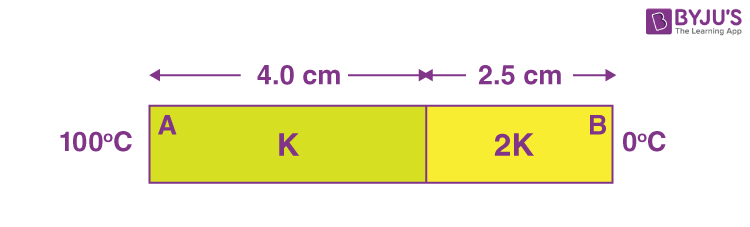Sol.

$$\begin{array}{l}\frac{L_1}{K_1A_1}+\frac{L_2}{K_2A_2}=\frac{L_1+L_2}{K_{eff}A_{eff}}\end{array}$$
$$\begin{array}{l}\Rightarrow \frac{4}{K}+\frac{2.5}{2K}=\frac{6.5}{K_{eff}}\end{array}$$
$$\begin{array}{l}\Rightarrow \frac{10.5}{2K}=\frac{6.5}{K_{eff}}\end{array}$$
$$\begin{array}{l}\Rightarrow K_{eff}=\frac{13K}{10.5}=\left ( 1+\frac{5}{21} \right )K\end{array}$$

⇒ α = 21

4. A body is performing simple harmonic with an amplitude of 10 cm. The velocity of the body was tripled by air Jet when it is at 5 cm from its mean position. The new amplitude of vibration is √x cm. The value of x is ___.

Sol.

$$\begin{array}{l}v=\omega\sqrt{A^2-y^2}\end{array}$$
$$\begin{array}{l}\Rightarrow 3\omega\sqrt{10^2-5^2}=\omega\sqrt{\left ( A’ \right )^2-5^2} \end{array}$$

⇒ 9 × 75 = (A′)2 – 25

$$\begin{array}{l}\Rightarrow A’=\sqrt{28\times25}~\textup{cm} \end{array}$$

x = 700

5. The variation of applied potential and current flowing through a given wire is shown in figure. The length of wire is 31.4 cm. The diameter of wire is measured as 2.4 cm. The resistivity of the given wire is measured as x × 10–3Ω cm. The value of x is____.

[Take π = 3.14]

Sol. Resistance = tan 45° = 1 Ω

$$\begin{array}{l}\Rightarrow 1=\frac{\rho l}{A} \end{array}$$
$$\begin{array}{l}\Rightarrow \rho=\frac{\pi\left ( 1.2~\text{cm} \right )^2}{31.4~\text{cm}}=1.44\times10^{-1}~\Omega \text{cm}\end{array}$$

x = 144

6. 300 cal. of heat is given to a heat engine and it rejects 225 cal. of heat. If source temperature is 227°C, then the temperature of sink will be _______°C.

Sol.

$$\begin{array}{l}\eta=\frac{W}{Q}=\frac{300-225}{300}\end{array}$$
$$\begin{array}{l}\Rightarrow \frac{75}{300}=1-\frac{T_L}{T_H}\end{array}$$
$$\begin{array}{l}\Rightarrow T_L=\frac{3}{4}~T_H=\frac{3}{4}\left ( 500 \right ) = 375\ K\end{array}$$

TL = 102°C

7.

$$\begin{array}{l}\sqrt{d_1}\ and\ \sqrt{d_2}\end{array}$$
are the impact parameters corresponding to scattering angles 60° and 90° respectively, when an α particle is approaching a gold nucleus. For d1 = xd2, the value of x will be _______.

Sol.

$$\begin{array}{l}\text{Impact parameter} \propto~\cot\frac{\theta}{2} \end{array}$$
$$\begin{array}{l}\Rightarrow\sqrt{\frac{d_1}{d_2}}=\frac{\sqrt{3}}{1}\end{array}$$

d1 = 3d2

x = 3

8. A transistor is used in an amplifier circuit in common emitter mode. If the base current changes by 100μA, it brings a change of 10mA in collector current. If the load resistance is 2kΩ and input resistance is 1kΩ, the value of power gain is x×104. The value of x is _______.

Sol. Power gain

$$\begin{array}{l}=\left [ \frac{\Delta i_C}{\Delta i_B} \right ]^2\times\frac{R_0}{R_i}\end{array}$$
$$\begin{array}{l}=\left [ \frac{10^{-2}}{10^{-4}} \right ]^2\times\frac{2}{1}\end{array}$$

= 2 × 104

x = 2

9. A parallel beam of light is allowed to fall on a transparent spherical globe of diameter 30 cm and refractive index 1.5. The distance from the centre of the globe at which the beam of light can converge is________ mm.

Sol.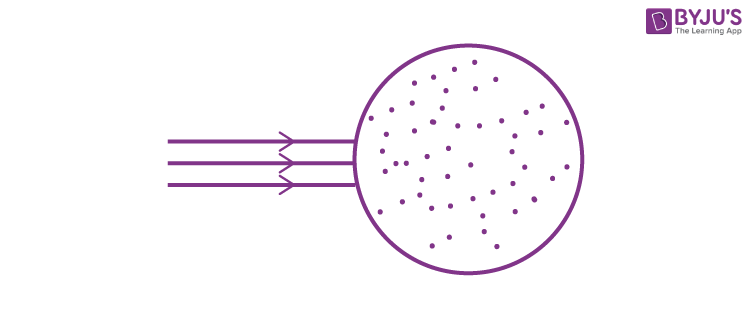1st refraction:

$$\begin{array}{l}\frac{1.5}{V_1}-0=\frac{0.5}{15} \end{array}$$

v1 = 45 cm

2nd refraction:

$$\begin{array}{l}\frac{1}{v_2}-\frac{1.5}{15}=\frac{-0.5}{-15}\end{array}$$
$$\begin{array}{l}\Rightarrow \frac{1}{v_2}=\frac{1}{30}+\frac{1}{10}\end{array}$$
$$\begin{array}{l}=\frac{4}{30}\end{array}$$

v2 = +7.5 cm

⇒ Distance from centre = 22.5 cm

10. For the network shown below, the value of VBVA is ________V.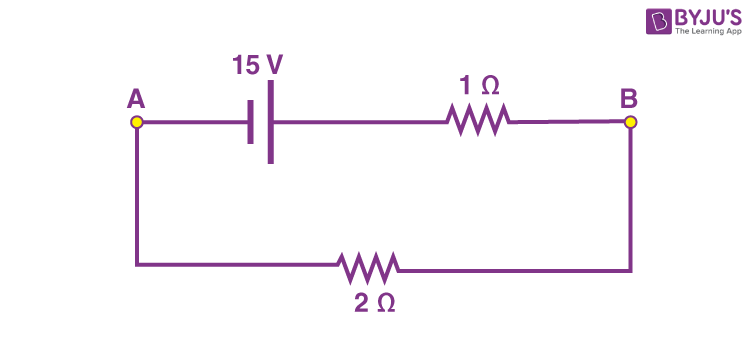Sol. VB– VA = i × 2

$$\begin{array}{l}=\frac{15}{1+2}\times2 \end{array}$$

VB– VA = 10 volts

### Download PDF of JEE Main 2022 June 29 Shift 1 Physics Paper & Solutions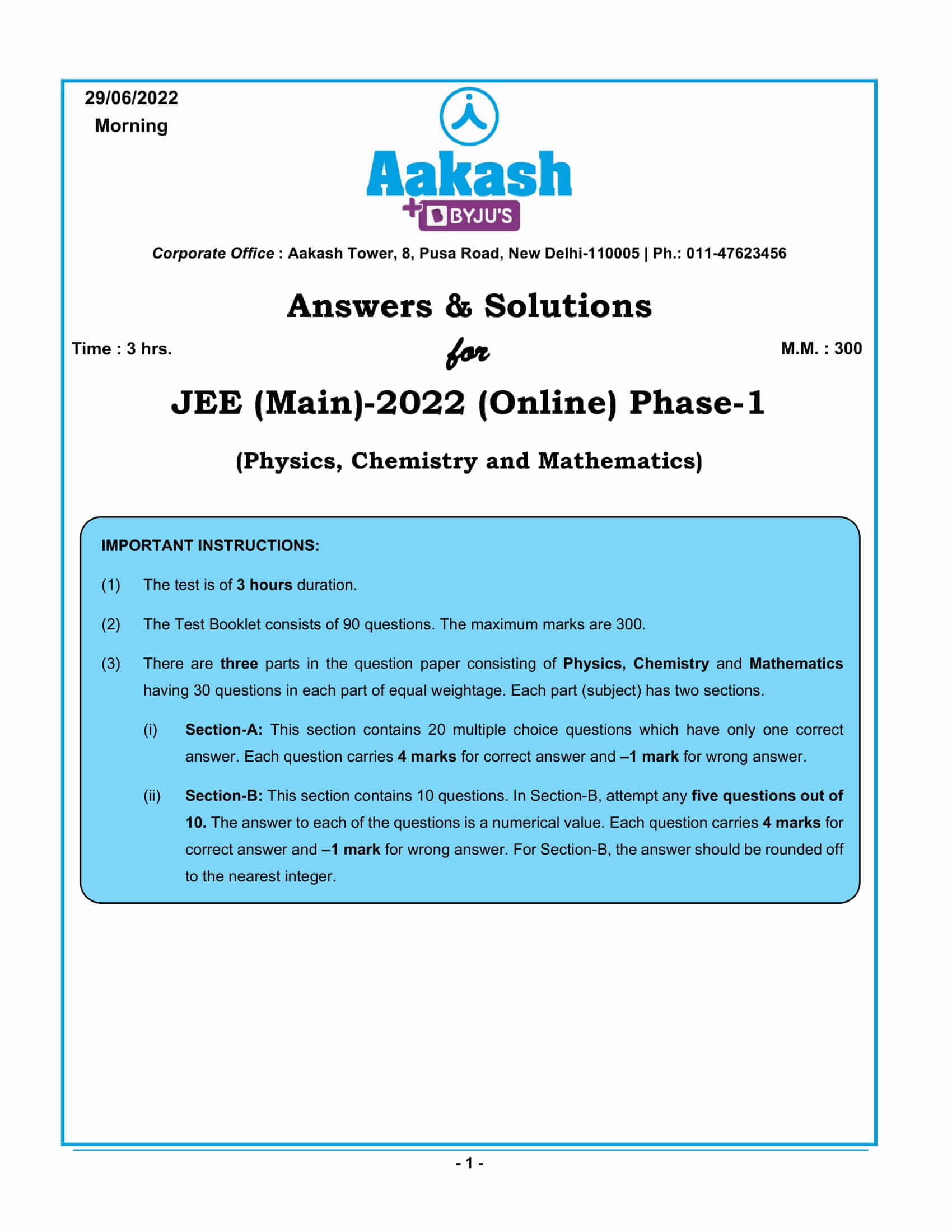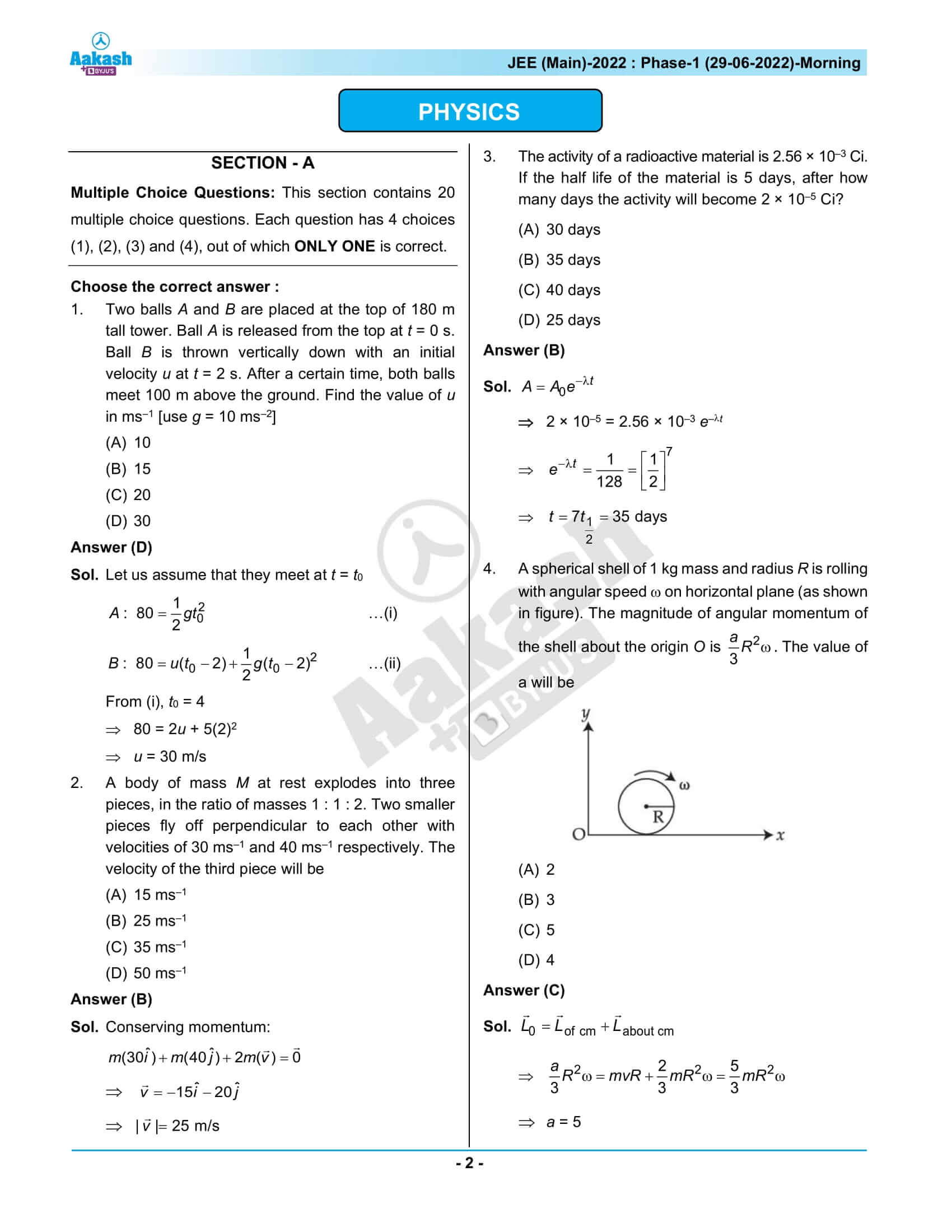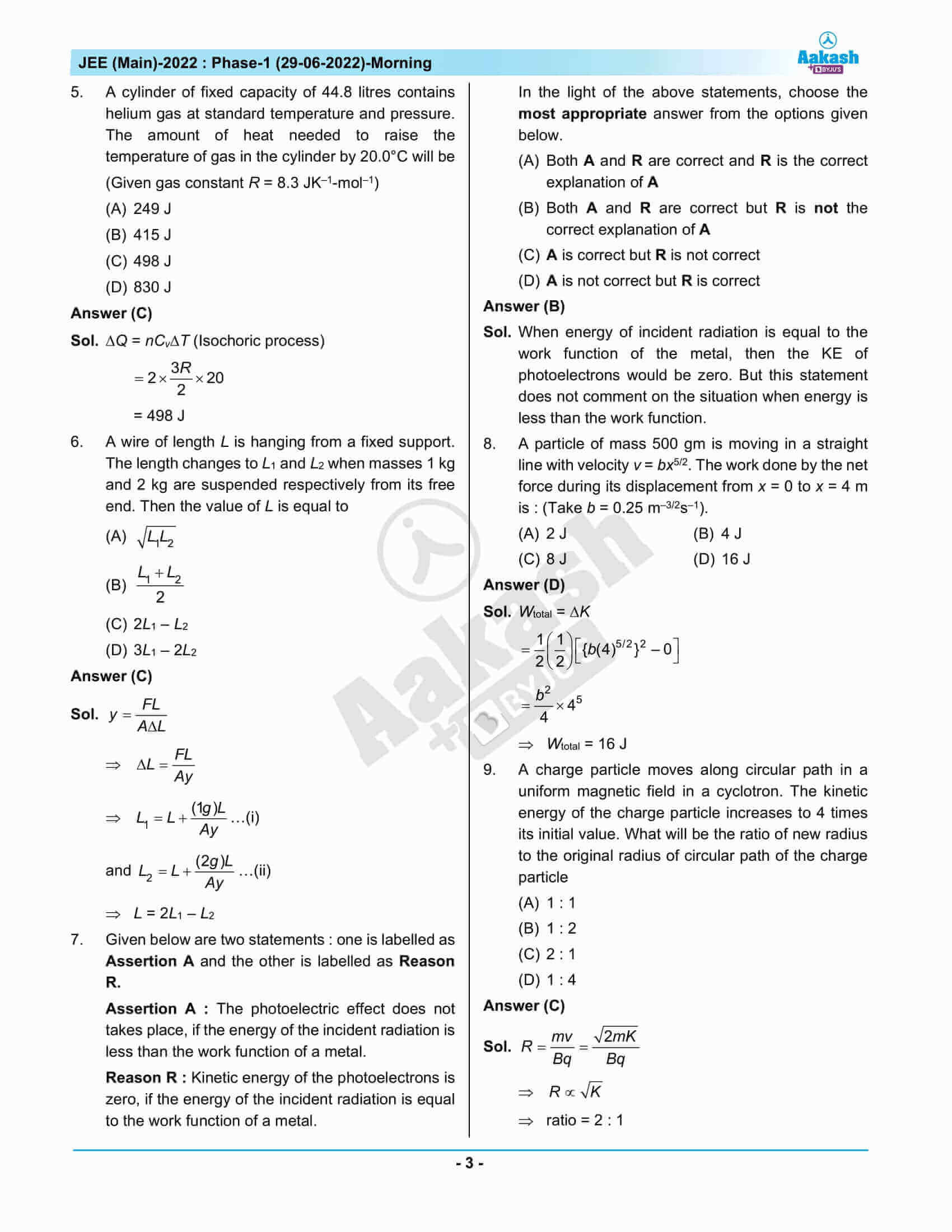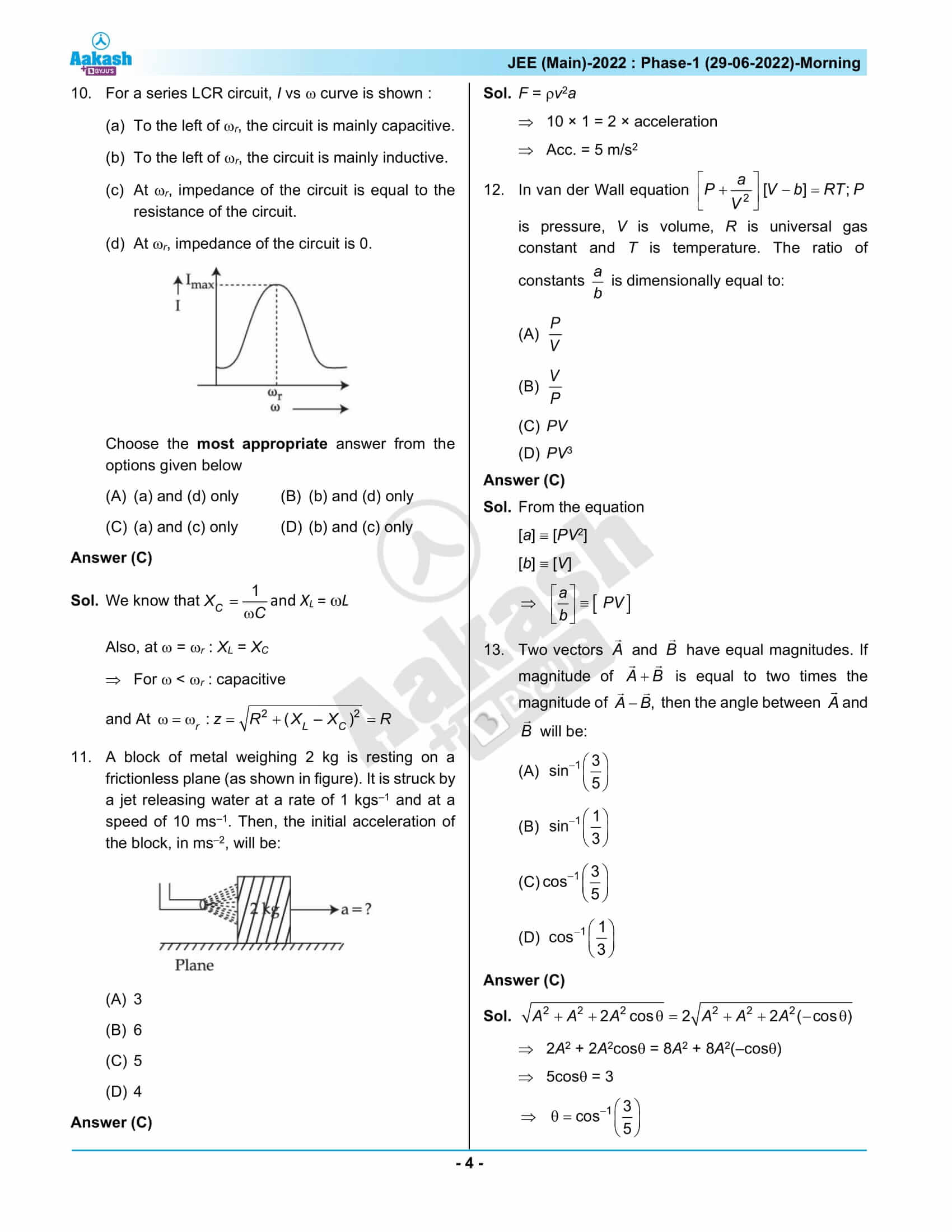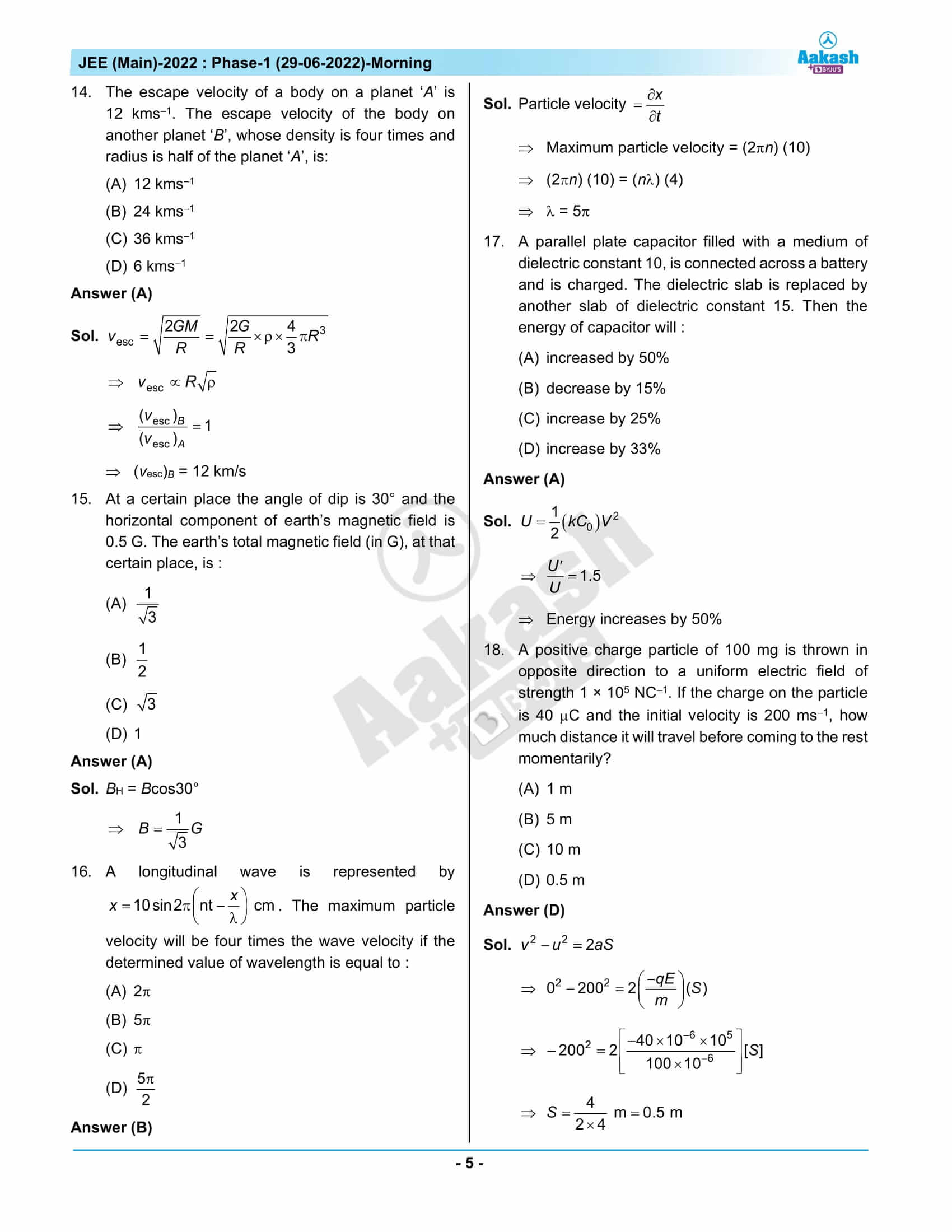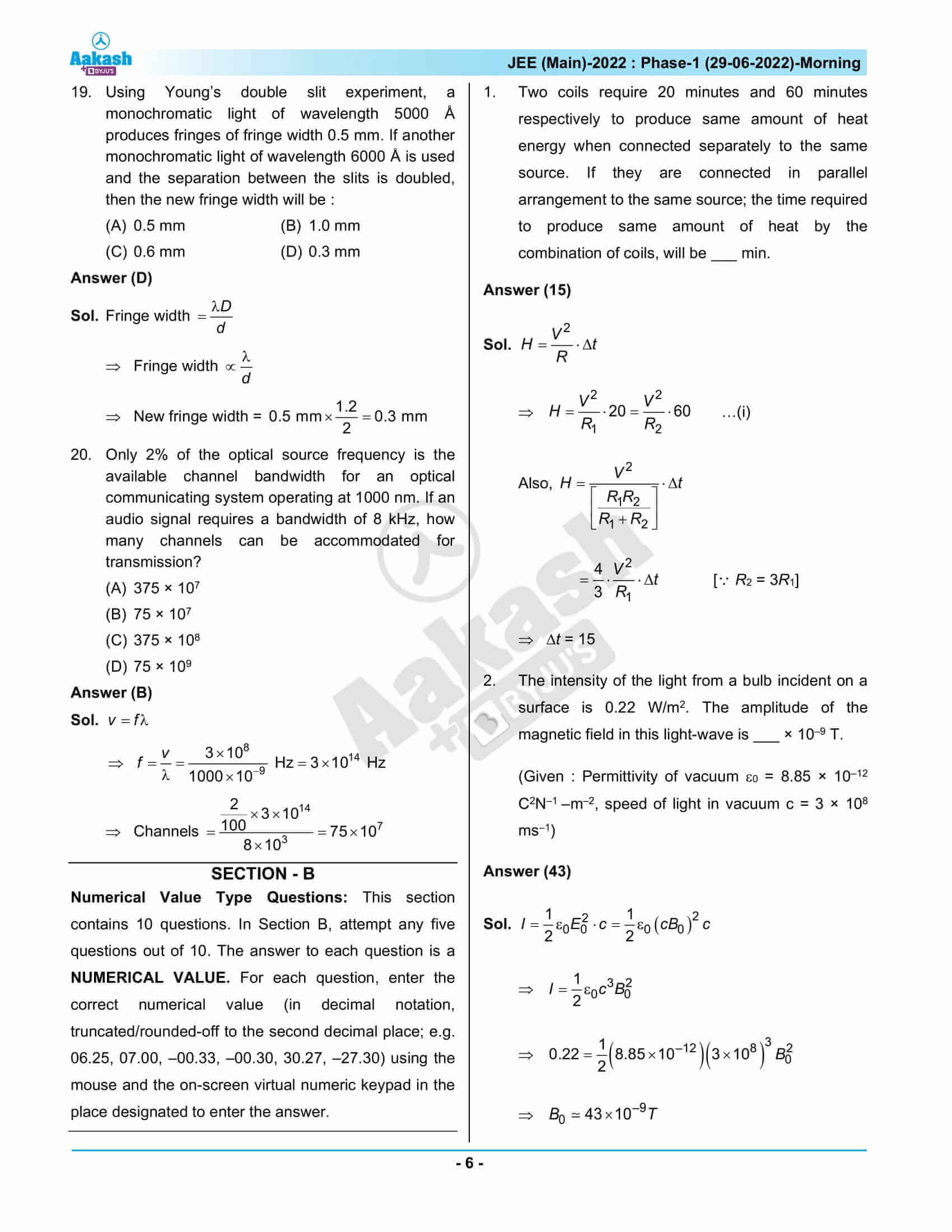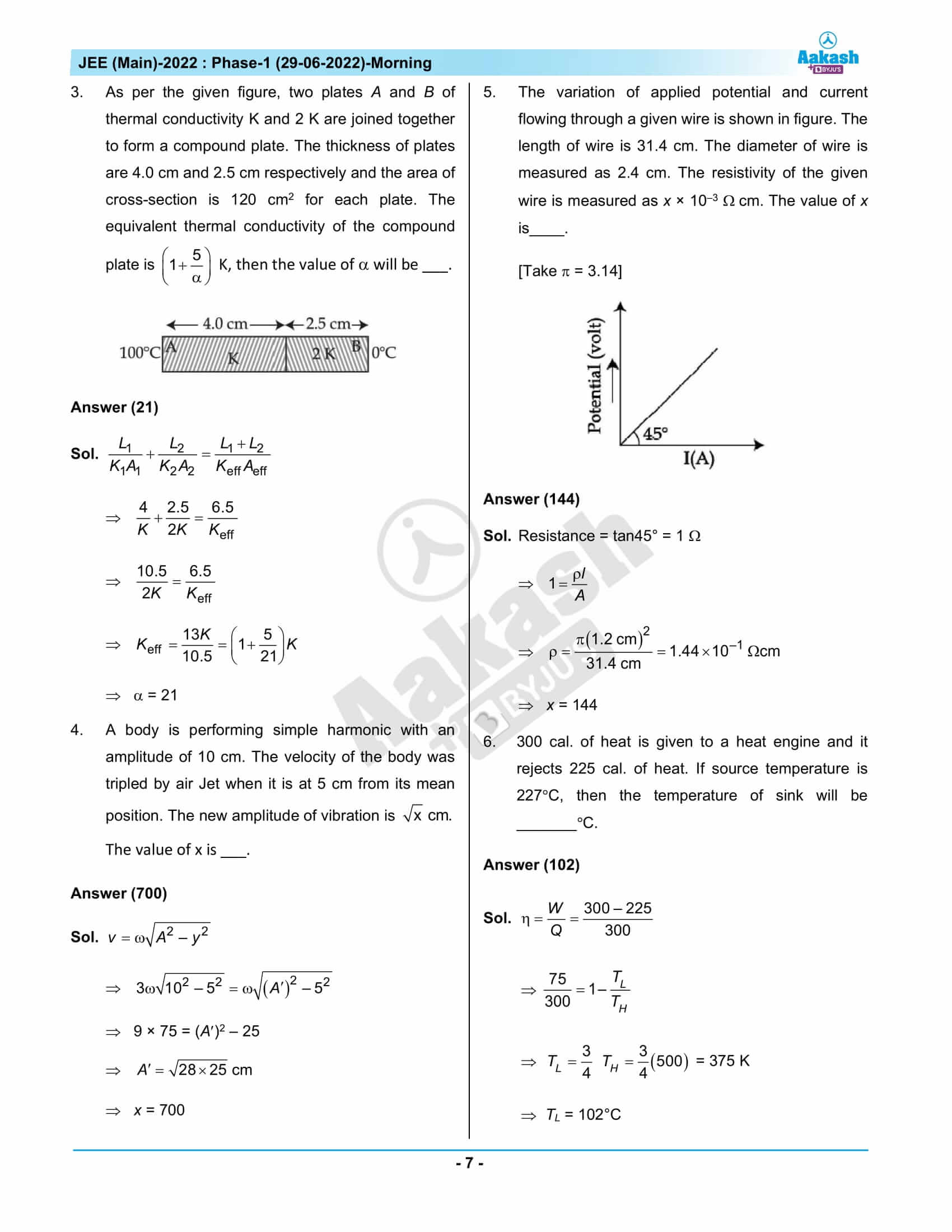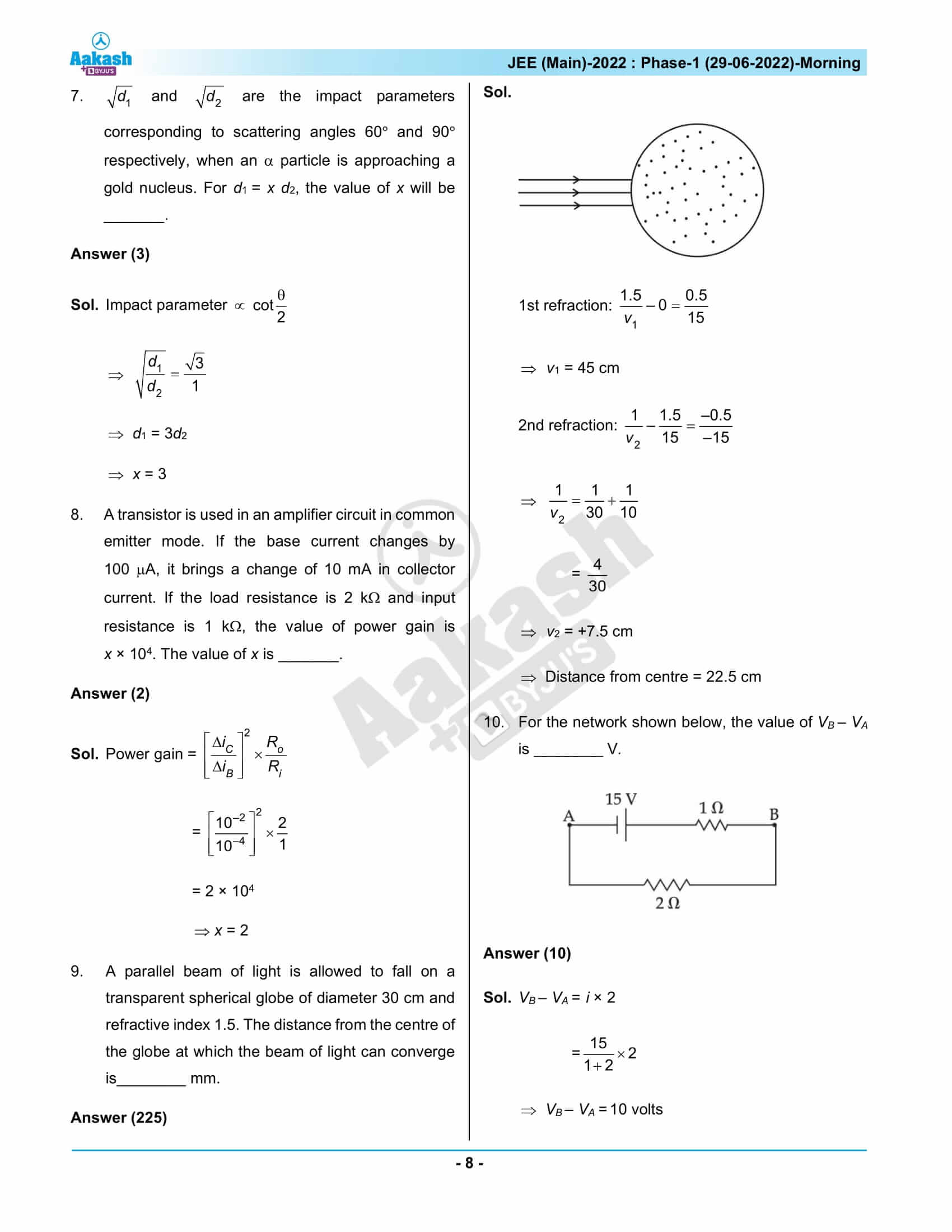## JEE Main 2022 June 29th Shift 1 Question Paper & Solutions

### JEE Main 2022 June 29 Shift 1 Question Paper – Physics Solutions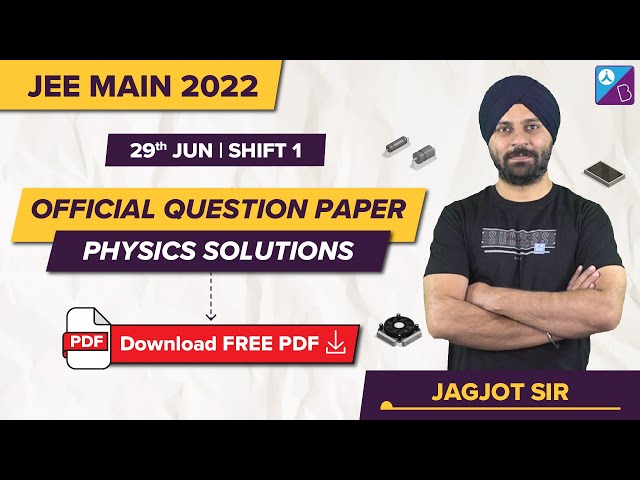### JEE Main 2022 June 29 Shift 1 Question Paper – Chemistry Solutions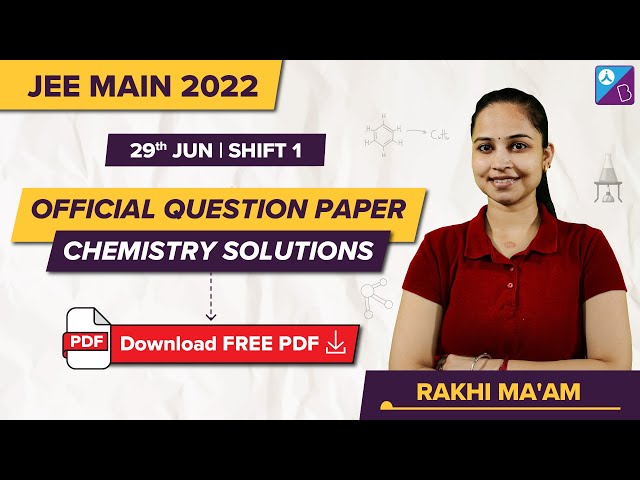### JEE Main 2022 June 29 Shift 1 Question Paper – Maths Solutions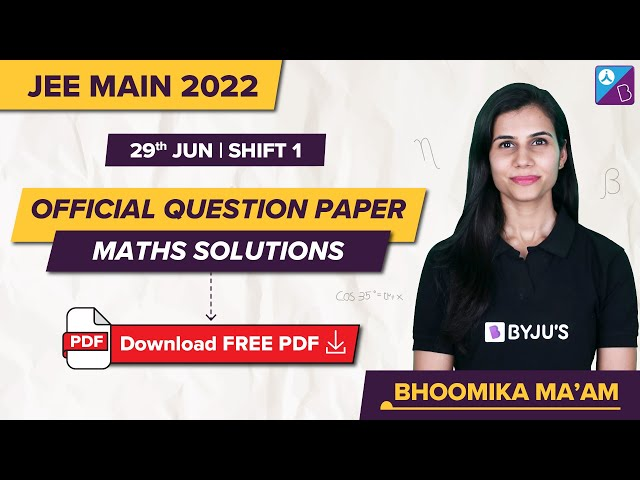## Frequently Asked Questions – FAQs

Q1

### What is the nature of the Physics questions asked in the JEE Main 2022 Question Paper conducted on the June 29th morning shift?

Most of the Physics questions were either easy or of moderate difficulty.

Q2

### Which subject questions were the easiest in JEE Main 2022 Question Paper conducted on the June 29th morning shift?

Physics was easier than Chemistry and Mathematics.

Q3

### What were the topics from which the Physics questions on the JEE Main 2022 June 29 Shift 1 question paper were taken?

Some of the topics included in the Physics are
Youngs Double Slit Experiment
Conservation of Momentum
Combination of Resistors – Series and Parallel
Motion under gravity
Photoelectric Effect and Wave Theory of Light
Escape Speed
Simple Harmonic Motion
Intensity of Electromagnetic Wave, etc.

Q4

### What was the difficulty level of the Physics questions asked in JEE Main 2022 Question Paper June 29 Shift 1 question paper?

The difficulty level of the Physics questions is 1.48 on a scale of 1 to 3.

Q5

### Which Physics chapters are included in JEE Main 2022 Question Paper June 29 Shift 1 from Class 12 syllabus?

The Physics chapters included from the Class 12 syllabus are:
Wave Optics
Current Electricity
Dual Nature of Radiation and Matter
Electromagnetic Waves
Alternating Current
Moving Charges and Magnetism
Electrostatic Potential and Capacitance
Electric Charges and Fields
Communication Systems
Ray Optics and Optical Instruments
Semiconductor Electronics: Materials, Devices and Simple Circuits
Magnetism and Matter